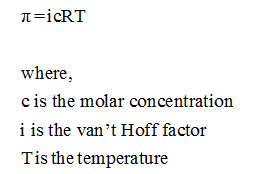# which will generate higher osmotic pressure at 298 K 1 L of a 0.250M cesium sulfate solution or 1L of a 0.220M phosphoric acid and why? assuming 100% dissociation into ions. Also please tell me how you know how many ions are formed...is there a math equation for that??

Question
1 views

which will generate higher osmotic pressure at 298 K 1 L of a 0.250M cesium sulfate solution or 1L of a 0.220M phosphoric acid and why? assuming 100% dissociation into ions. Also please tell me how you know how many ions are formed...is there a math equation for that??

check_circle

Step 1

Osmotic pressure is calculated by using the equation,Step 2

Given that the molarity of Cesium sulfate solution is 0.250 M at 298 K and molarity of phosphoric acid is 0.220 M at the same temperature. Van’t Hoff factor can be defines as the number of ions formed from one molecule of the solute when 100% dissociation of solute occurs. In-order to find out the number of ions formed, the dis...

### Want to see the full answer?

See Solution

#### Want to see this answer and more?

Solutions are written by subject experts who are available 24/7. Questions are typically answered within 1 hour.*

See Solution
*Response times may vary by subject and question.
Tagged in